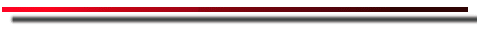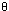Practicing Using the CatapultThe Catapult Applet requires a browser that can run Java

1. Turn off the air resistance. Making sure you change only one variable (ie. initial velocity) at a time, try to get some projectiles to hit the traget (at a range of 100 meters). Before firing each projectile, try to predict the range using:

R = vo2 sin(2)/g

where vo is the launch speed,is the launch angle (from horizontal), and g is the gravitational acceleration (9.8 meters/second2).
Does your computed range match the one on screen? The final range you achieved appears on the status line at the bottom of your browser. (NOTE: Do not adjust the launch height).

2. Next, turn on the air resistance and vary the terminal velocity. Use the equation above to compute the expected range (without air resistance). Compare the expected range to the observed range. Explain why they are different.

3. Turn off the air resistance again. Now vary gravity and compare the trajectories (this is obviously something you could not do on earth, at least easily).

4. Now, turn on the air resistance one last time. Vary only the time step in incriments of 0.2 until you get to a time step of 1. What do you notice?

The time step is the incriment over which the equations are calculated. The smaller the time step the smoother, and more accurate the simulation. Of course, using smaller time steps also means many more calculations. If you had to do this by hand it would take a very long time, good thing you have a computer close by.

Go Back To the Catapult Homepage